# 6th-8th Grade Geometry: Circular Arcs & Measurement Chapter Exam

Exam Instructions:

Choose your answers to the questions and click 'Next' to see the next set of questions. You can skip questions if you would like and come back to them later with the yellow "Go To First Skipped Question" button. When you have completed the practice exam, a green submit button will appear. Click it to see your results. Good luck!

### Page 1

#### Question 3 3. If AB and CB are tangent segments, what is the length of CB?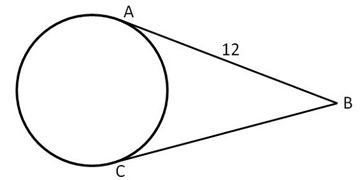#### Question 5 5. In the pictured circle, the measure of angle ACB is 22 degrees and the measure of angle BXD is 120 degrees. What is the measure of angle CAD?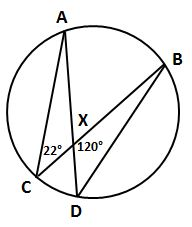### Page 2

#### Question 6 6. If the area of the square is 64, what is the area of the circle, rounded to the nearest whole number?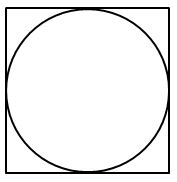#### Question 7 7. If angle ACB is 34 degrees, what is the measure of angle ADB?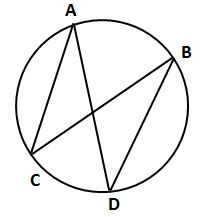#### Question 10 10. In the picture below, if arc BD is 168 degrees and arc BC is 70 degrees, what is the measure of angle BAD?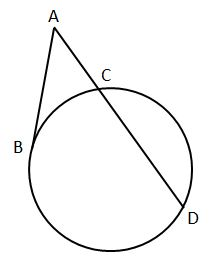### Page 3

#### Question 11 11. In the picture below, if arc CE is 172 degrees and arc BD is 80 degrees, what is the measure of angle CAE?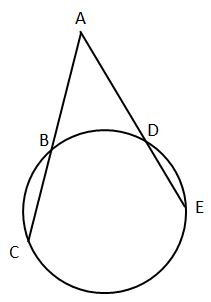#### Question 12 12. If angle AOB is 40 degrees, what is the measure of angle ACB?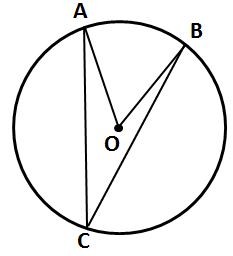#### Question 15 15. In the picture below, if AC is 14 and AB is 10, what is the length of AD?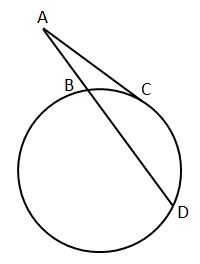### Page 4

#### Question 16 16. In the picture below, if AB is 7, BC is 19 and AD is 8, what is the length of DE?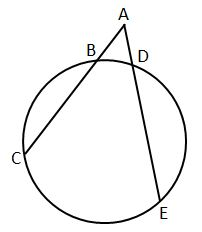#### Question 17 17. If arc BC is 84 degrees, what is the measure of angle ACB?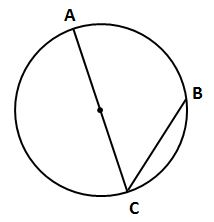#### Question 18 18. In the picture below, if arc AB is 82 degrees, what is the measure of angle BAC?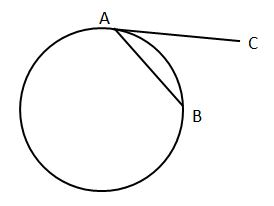#### Question 19 19. If the measure of central angle AOB is 62 degrees, what is the measure of inscribed angle ACB?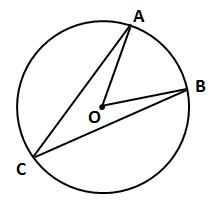#### Question 20 20. In the picture below, if major arc BC is 258 degrees and minor arc BC is 102 degrees, what is the measure of angle BAC?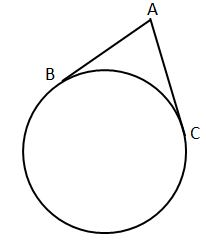### Page 5

#### Question 23 23. If angle ACB is 47 degrees, what is the measure of angle AOB?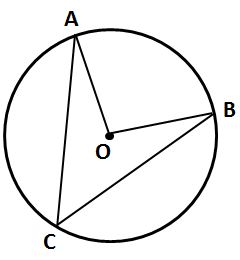#### Question 24 24. In the picture below, if AE is 10, AB is 14 and ED is 16, what is the length of CE?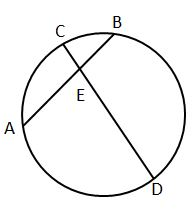### Page 6

#### Question 28 28. If angle OAB is 45 degrees, what is the measure of angle AOB?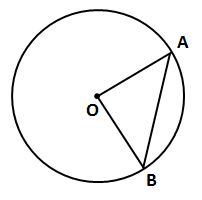#### 6th-8th Grade Geometry: Circular Arcs & Measurement Chapter Exam Instructions

Choose your answers to the questions and click 'Next' to see the next set of questions. You can skip questions if you would like and come back to them later with the yellow "Go To First Skipped Question" button. When you have completed the practice exam, a green submit button will appear. Click it to see your results. Good luck!

Support